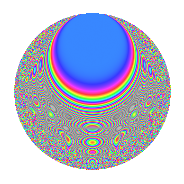# Properties

 Label 8005.2.a.fLevel 8005 Weight 2 Character orbit 8005.a Self dual Yes Analytic conductor 63.920 Analytic rank 1 Dimension 127 CM No

# Related objects

## Newspace parameters

 Level: $$N$$ = $$8005 = 5 \cdot 1601$$ Weight: $$k$$ = $$2$$ Character orbit: $$[\chi]$$ = 8005.a (trivial)

## Newform invariants

 Self dual: Yes Analytic conductor: $$63.920246818$$ Analytic rank: $$1$$ Dimension: $$127$$ Fricke sign: $$1$$ Sato-Tate group: $\mathrm{SU}(2)$

## $q$-expansion

The dimension is sufficiently large that we do not compute an algebraic $$q$$-expansion, but we have computed the trace expansion.

 $$\operatorname{Tr}(f)(q) =$$ $$127q$$ $$\mathstrut -\mathstrut 6q^{2}$$ $$\mathstrut -\mathstrut 18q^{3}$$ $$\mathstrut +\mathstrut 114q^{4}$$ $$\mathstrut -\mathstrut 127q^{5}$$ $$\mathstrut -\mathstrut 20q^{6}$$ $$\mathstrut +\mathstrut 28q^{7}$$ $$\mathstrut -\mathstrut 18q^{8}$$ $$\mathstrut +\mathstrut 101q^{9}$$ $$\mathstrut +\mathstrut O(q^{10})$$ $$\operatorname{Tr}(f)(q) =$$ $$127q$$ $$\mathstrut -\mathstrut 6q^{2}$$ $$\mathstrut -\mathstrut 18q^{3}$$ $$\mathstrut +\mathstrut 114q^{4}$$ $$\mathstrut -\mathstrut 127q^{5}$$ $$\mathstrut -\mathstrut 20q^{6}$$ $$\mathstrut +\mathstrut 28q^{7}$$ $$\mathstrut -\mathstrut 18q^{8}$$ $$\mathstrut +\mathstrut 101q^{9}$$ $$\mathstrut +\mathstrut 6q^{10}$$ $$\mathstrut -\mathstrut 45q^{11}$$ $$\mathstrut -\mathstrut 30q^{12}$$ $$\mathstrut -\mathstrut 53q^{14}$$ $$\mathstrut +\mathstrut 18q^{15}$$ $$\mathstrut +\mathstrut 84q^{16}$$ $$\mathstrut -\mathstrut 36q^{17}$$ $$\mathstrut -\mathstrut 10q^{18}$$ $$\mathstrut -\mathstrut 49q^{19}$$ $$\mathstrut -\mathstrut 114q^{20}$$ $$\mathstrut -\mathstrut 48q^{21}$$ $$\mathstrut +\mathstrut 13q^{22}$$ $$\mathstrut -\mathstrut 29q^{23}$$ $$\mathstrut -\mathstrut 63q^{24}$$ $$\mathstrut +\mathstrut 127q^{25}$$ $$\mathstrut -\mathstrut 55q^{26}$$ $$\mathstrut -\mathstrut 75q^{27}$$ $$\mathstrut +\mathstrut 44q^{28}$$ $$\mathstrut -\mathstrut 45q^{29}$$ $$\mathstrut +\mathstrut 20q^{30}$$ $$\mathstrut -\mathstrut 49q^{31}$$ $$\mathstrut -\mathstrut 32q^{32}$$ $$\mathstrut -\mathstrut 8q^{33}$$ $$\mathstrut -\mathstrut 52q^{34}$$ $$\mathstrut -\mathstrut 28q^{35}$$ $$\mathstrut +\mathstrut 44q^{36}$$ $$\mathstrut +\mathstrut 36q^{37}$$ $$\mathstrut -\mathstrut 65q^{38}$$ $$\mathstrut -\mathstrut 52q^{39}$$ $$\mathstrut +\mathstrut 18q^{40}$$ $$\mathstrut -\mathstrut 66q^{41}$$ $$\mathstrut -\mathstrut 18q^{42}$$ $$\mathstrut -\mathstrut 5q^{43}$$ $$\mathstrut -\mathstrut 93q^{44}$$ $$\mathstrut -\mathstrut 101q^{45}$$ $$\mathstrut -\mathstrut 25q^{46}$$ $$\mathstrut -\mathstrut 32q^{47}$$ $$\mathstrut -\mathstrut 54q^{48}$$ $$\mathstrut +\mathstrut 77q^{49}$$ $$\mathstrut -\mathstrut 6q^{50}$$ $$\mathstrut -\mathstrut 102q^{51}$$ $$\mathstrut -\mathstrut 13q^{52}$$ $$\mathstrut -\mathstrut 67q^{53}$$ $$\mathstrut -\mathstrut 53q^{54}$$ $$\mathstrut +\mathstrut 45q^{55}$$ $$\mathstrut -\mathstrut 158q^{56}$$ $$\mathstrut +\mathstrut 16q^{57}$$ $$\mathstrut +\mathstrut 35q^{58}$$ $$\mathstrut -\mathstrut 213q^{59}$$ $$\mathstrut +\mathstrut 30q^{60}$$ $$\mathstrut -\mathstrut 62q^{61}$$ $$\mathstrut -\mathstrut 33q^{62}$$ $$\mathstrut +\mathstrut 59q^{63}$$ $$\mathstrut +\mathstrut 34q^{64}$$ $$\mathstrut -\mathstrut 60q^{66}$$ $$\mathstrut +\mathstrut 10q^{67}$$ $$\mathstrut -\mathstrut 94q^{68}$$ $$\mathstrut -\mathstrut 93q^{69}$$ $$\mathstrut +\mathstrut 53q^{70}$$ $$\mathstrut -\mathstrut 118q^{71}$$ $$\mathstrut -\mathstrut 24q^{72}$$ $$\mathstrut +\mathstrut 35q^{73}$$ $$\mathstrut -\mathstrut 107q^{74}$$ $$\mathstrut -\mathstrut 18q^{75}$$ $$\mathstrut -\mathstrut 98q^{76}$$ $$\mathstrut -\mathstrut 93q^{77}$$ $$\mathstrut +\mathstrut 21q^{78}$$ $$\mathstrut -\mathstrut 64q^{79}$$ $$\mathstrut -\mathstrut 84q^{80}$$ $$\mathstrut +\mathstrut 15q^{81}$$ $$\mathstrut +\mathstrut 15q^{82}$$ $$\mathstrut -\mathstrut 187q^{83}$$ $$\mathstrut -\mathstrut 118q^{84}$$ $$\mathstrut +\mathstrut 36q^{85}$$ $$\mathstrut -\mathstrut 126q^{86}$$ $$\mathstrut -\mathstrut 53q^{87}$$ $$\mathstrut +\mathstrut 15q^{88}$$ $$\mathstrut -\mathstrut 138q^{89}$$ $$\mathstrut +\mathstrut 10q^{90}$$ $$\mathstrut -\mathstrut 138q^{91}$$ $$\mathstrut -\mathstrut 86q^{92}$$ $$\mathstrut +\mathstrut 23q^{93}$$ $$\mathstrut -\mathstrut 60q^{94}$$ $$\mathstrut +\mathstrut 49q^{95}$$ $$\mathstrut -\mathstrut 92q^{96}$$ $$\mathstrut +\mathstrut 9q^{97}$$ $$\mathstrut -\mathstrut 67q^{98}$$ $$\mathstrut -\mathstrut 147q^{99}$$ $$\mathstrut +\mathstrut O(q^{100})$$

## Embeddings

For each embedding $$\iota_m$$ of the coefficient field, the values $$\iota_m(a_n)$$ are shown below.

For more information on an embedded modular form you can click on its label.

Label $$a_{2}$$ $$a_{3}$$ $$a_{4}$$ $$a_{5}$$ $$a_{6}$$ $$a_{7}$$ $$a_{8}$$ $$a_{9}$$ $$a_{10}$$
1.1 −2.78690 −1.15761 5.76683 −1.00000 3.22614 5.01285 −10.4978 −1.65994 2.78690
1.2 −2.73456 1.66625 5.47781 −1.00000 −4.55646 2.63613 −9.51029 −0.223607 2.73456
1.3 −2.71909 −0.177103 5.39346 −1.00000 0.481560 0.324903 −9.22712 −2.96863 2.71909
1.4 −2.69002 2.05113 5.23619 −1.00000 −5.51758 −1.38538 −8.70541 1.20715 2.69002
1.5 −2.58596 −1.41933 4.68721 −1.00000 3.67034 −1.12236 −6.94904 −0.985504 2.58596
1.6 −2.57363 −3.19039 4.62356 −1.00000 8.21088 4.45582 −6.75206 7.17859 2.57363
1.7 −2.55017 −2.04848 4.50339 −1.00000 5.22398 0.317242 −6.38407 1.19626 2.55017
1.8 −2.54111 −2.08826 4.45724 −1.00000 5.30651 2.18201 −6.24412 1.36084 2.54111
1.9 −2.51650 0.753102 4.33277 −1.00000 −1.89518 3.99606 −5.87042 −2.43284 2.51650
1.10 −2.51085 1.02778 4.30438 −1.00000 −2.58061 −1.85755 −5.78596 −1.94366 2.51085
1.11 −2.50760 2.92815 4.28808 −1.00000 −7.34263 −1.39021 −5.73759 5.57405 2.50760
1.12 −2.43228 1.96248 3.91599 −1.00000 −4.77331 4.23453 −4.66022 0.851341 2.43228
1.13 −2.42159 1.03210 3.86412 −1.00000 −2.49933 −3.82183 −4.51414 −1.93477 2.42159
1.14 −2.40828 3.08031 3.79980 −1.00000 −7.41825 3.21201 −4.33442 6.48832 2.40828
1.15 −2.24882 2.37302 3.05719 −1.00000 −5.33649 −0.662910 −2.37743 2.63123 2.24882
1.16 −2.17575 0.162649 2.73390 −1.00000 −0.353884 −3.43107 −1.59678 −2.97355 2.17575
1.17 −2.15149 −2.91656 2.62891 −1.00000 6.27495 1.67048 −1.35310 5.50631 2.15149
1.18 −2.10573 −1.72602 2.43410 −1.00000 3.63453 0.366521 −0.914097 −0.0208610 2.10573
1.19 −2.10003 −0.379559 2.41011 −1.00000 0.797083 −3.17706 −0.861245 −2.85594 2.10003
1.20 −2.09752 −1.19582 2.39960 −1.00000 2.50825 4.08912 −0.838178 −1.57003 2.09752
See next 80 embeddings (of 127 total)
 $$n$$: e.g. 2-40 or 990-1000 Embeddings: e.g. 1-3 or 1.127 Significant digits: Format: Complex embeddings Normalized embeddings Satake parameters Satake angles

## Inner twists

This newform does not have CM; other inner twists have not been computed.

## Atkin-Lehner signs

$$p$$ Sign
$$5$$ $$1$$
$$1601$$ $$1$$

## Hecke kernels

This newform can be constructed as the intersection of the kernels of the following linear operators acting on $$S_{2}^{\mathrm{new}}(\Gamma_0(8005))$$:

 $$T_{2}^{127} + \cdots$$ $$T_{3}^{127} + \cdots$$